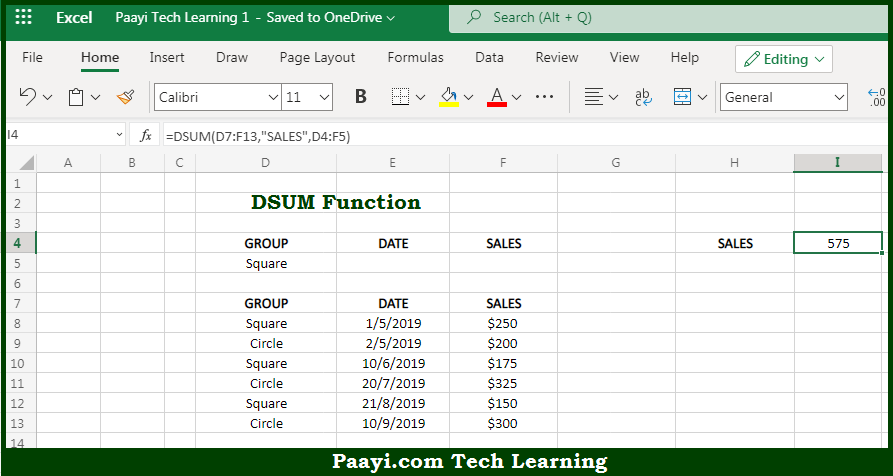# Learn How to Use Microsoft Excel DSUM Function

Written by | 0 Comments | 695 Views

In this article, you will learn how to use the Microsoft Excel DSUM function and its prime function in Microsoft Excel. You will also get to know the Microsoft Excel DSUM function return value and syntax with the help of some examples.

Microsoft Excel DSUM Function

The main purpose of the Microsoft Excel DSUM function is to get the sum from the matching records in the database. That implies, with the help of the DSUM function you can able to return the sum of values from a set of records that match criteria. It should be noted that the values to sum are extracted from a given field in the database. So, with the help of the DSUM function, you can able to get the sum from the matching records in the database.

Return Value of DSUM Function

The return value will be the calculated sum.

Syntax of DSUM Function

=DSUM(database, field, criteria)

Where the arguments:

• database: This is the database range including headers.
• field: This is a field name or index to count optional.
• criteria: This is the criteria range including headers.

How to Use Microsoft Excel DSUM Function?So we know that Microsoft Excel DSUM function you can able to get the sum from the matching records in the database. That implies, with the help of the DSUM function you can able to return the sum of values from a set of records that match criteria. It should be noted that the values to sum are extracted from a given field in the database. So, with the help of the DSUM function, you can able to get the sum from the matching records in the database.